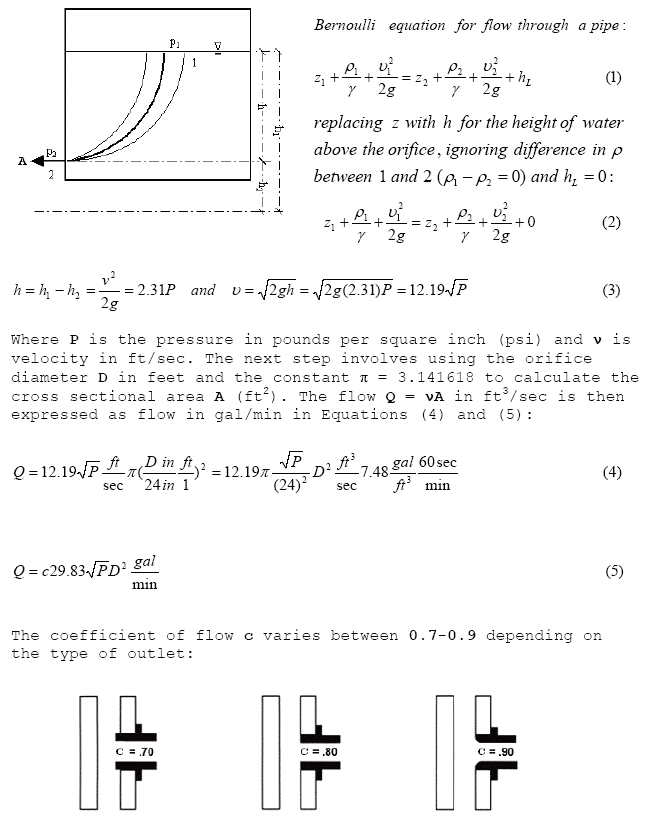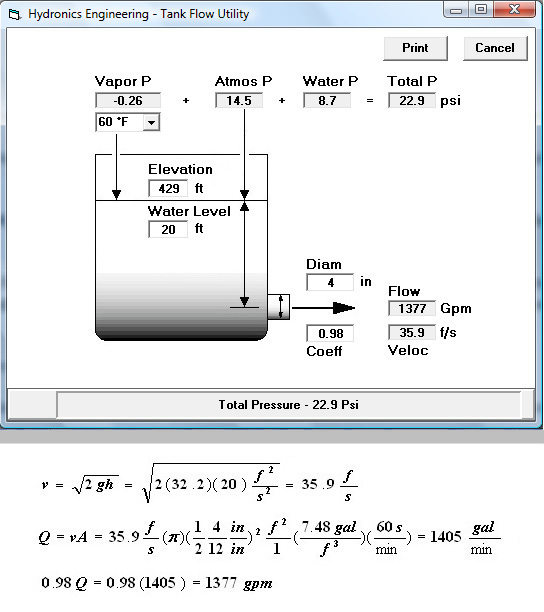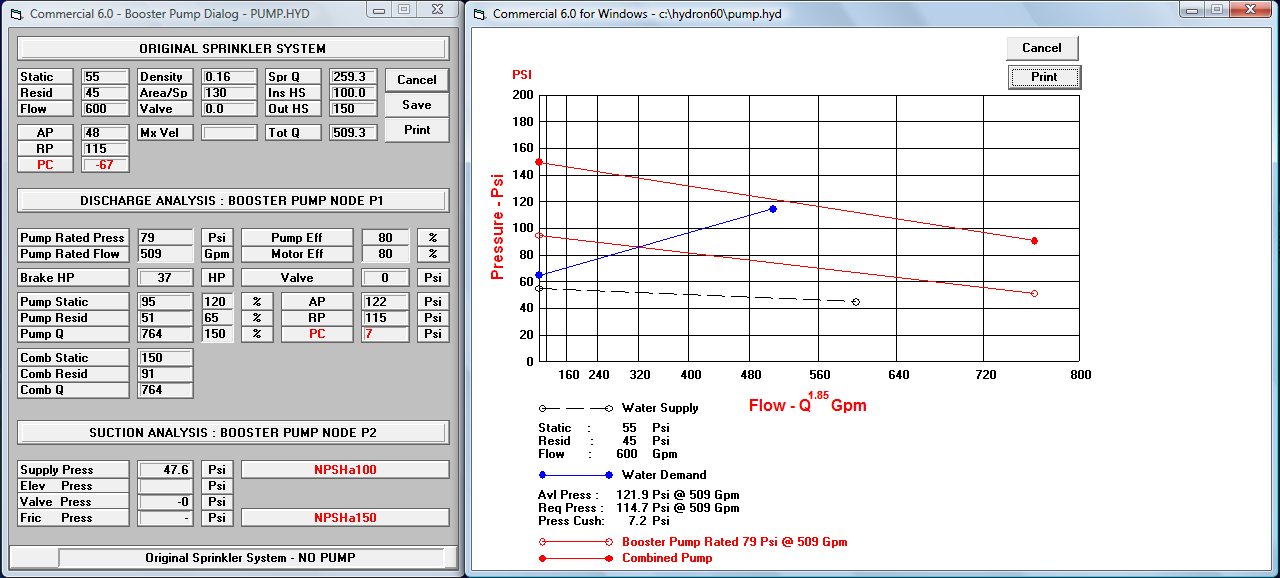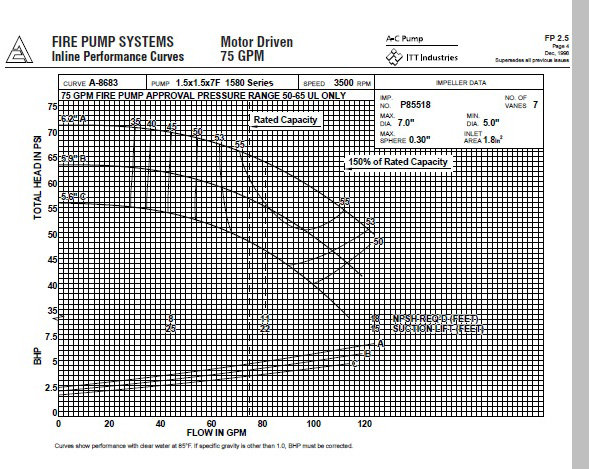Fire Sprinkler Hydraulic Calculation and Design Software.
 HomeContact

 Hydraulics and Fluid Mechanics Bernoulli Equation Hazen-Williams Equation Darcy-Weisbach Equation Moody Diagram Hydrant Flow Equation Water Tank Hydraulics Booster Pump Hydraulics Pump Curves Fire Sprinkler K-Factors Hydraulic Simulation Models Hydraulic Calculations and Fire Sprinkler Design Computers as Hydraulic Models PDF Documents Links to Articles Glossary

# Hydraulics and Fluid Mechanics

Hydraulics and Fluid Mechanics is that branch of applied mechanics dealing with the behavior of fluids at rest and in motion. Here is a synopsis of important concepts and ideas:
• Water exerts hydrostatic pressure because it weighs 62.4 lbs per cubic foot i.e. a container 1 foot long by 1 foot wide and 1 foot deep filled with water exerts 62.4/144 = 0.4333 psi at the base of the container. Does that sound familiar? So a 100 foot column of water exerts a pressure of 43.3 psi at it's base. Conversely a pressure of 43.3 psi is equivalent to 43.3(1/0.43333) = 2.3143.31 = 100 ft head of water. More on this later in connection with the Toricelli equation.

• Water exerts elevation pressure by virtue of it's height above a given datum e.g. sea level. A 50 foot deep tank filled with water and located on a hill 50 foot above sea level, would exert the same pressure as the 100 foot column of water i.e. 43.3 psi. That makes hill tops ideal for locating water tanks.

• Water flows in response to velocity pressure (v 2/2g) where v is the velocity in ft/sec and g is 32.2 ft/sec2 or the constant of gravitational acceleration. The pitot pressure from a hydrant flow test is a direct measure of the velocity pressure as expressed by Toricelli's Law:
h = n 2/2g = 2.31P ft
where:
h is a height of water (ft) .
v is the velocity of flow (ft/sec).
g is the constant of gravitational acceleration, equal to 32.2 ft/sec2.
P is the pitot pressure (psi).

Using the Bernoulli equation and Toricelli's Law, the flow may be expressed as a function of pressure P and outlet diameter D as shown in Figure 2.

• The Bernoulli equation ties all the different elements of pressure together from the source to the most remote sections of the sprinkler system. We must assume that the equations for continuity, steady state and impulse momentum apply i.e. ideal conditions that are not complicated by the effects of turbulence.

• Friction losses occur as water flows through fire sprinkler piping. Part of the friction loss is from direct contact with the interior of the pipe, fittings and devices such as valves. Part of it is due to turbulence within the fluid. The smoother the pipe the lower the friction loss. The Hazen-Williams equation is simple to use and works very well in estimating friction loss under a variety of flow conditions. The Darcy-Weisbach equation is better at estimating friction losses when flow is turbulent. However, it is computationally more complicated and yields only a minor improvement in accuracy over the Hazen-Williams equation.

• The flow from a discharging sprinkler is estimated by multiplying the sprinkler nozzle coefficient k by the square root of the pressure at the nozzle i.e. Q = kÖP = kP0.5, where Q is the flow (gpm), k is the sprinkler nozzle coefficient or K-Factor in dimensionless units and P is the sprinkler nozzle pressure (psi).

• A hydraulic calculation is a physical accounting system, very much like a checkbook, that balances energy income and expenditure. The Bernoulli equation is a mathematical expression of that balance.

### The Bernoulli Equation

The physical principles governing flow in pressure conduits was developed by Daniel Bernoulli (1700-1782) and published in his book Hydrodynamica in 1738. The Bernoulli equation is used to analyze fluid flow along a streamline from point 1 to point 2 and is expressed as:
z1 + r1/g + n12/2g = z2 + r2/g + n22/2g + hL
where:
z1 & z2 are the elevations at locations 1 and 2, also called the elevation head (ft).
r1 & r2 are the pressures at locations 1 and 2 (lbs/ft2).
g  is the specific density of water (62.4 lbs/ft3. )
n1 & n2 are the velocities at locations 1 and 2 (ft/sec).
g is the constant of gravitational acceleration (32.2 ft/sec2. )
hL is the Darcy-Weisbach estimate of headloss due to friction (ft).

Bernoulli was a friend and intellectual rival of legendary physicist Sir Isaac Newton. J.L. Lagrange (1736-1813) was the first to present the modern form of the equation in his book Mechanique Analytique published in 1788. Lagrange also listed the assumptions required for the Bernoulli equation to work. The Bernoulli equation assumes that the fluid and device meet 4 Lagrangian assumptions:
1. The fluid is incompressible
2. The fluid is inviscid
4. The flow is along a streamline
Most liquids meet the incompressible assumption and many gases can even be treated as incompressible if their density varies only slightly along a streamline from point 1 to point 2. The steady flow requirement is usually not too hard to achieve for situations typically analyzed by the Bernoulli equation. Steady flow means that the flow rate (i.e. discharge) does not vary with time. The inviscid fluid requirement implies that the fluid has no viscosity. All fluids have viscosity although viscous effects are negligible over short distances. The Bernoulli equation is important because it incorporates the major parameteric components used to describe and analyze the flow of any fluid through pipes or conduits under pressure.

In the Bernoulli equation, fluid flow occurs in response to differences in pressure between two points in a flow stream. Since our experience and intuition often confirms that water tends to flow downhill, the difference in elevation z (ft), between two points in the flow stream is the first component. The fluid column pressure r (lbs/ft2) is the second component. It is divided by the specific weight of the fluid g (62.4 lbs/ft3), to make it consistent with the linear units used in expressing the elevation component. The sum of the two is referred to as the piezometric head or the hydraulic grade line. The third component in the Bernoulli equation is velocity pressure n 2/2g. The sum of the three components, the total head available to the fluid, is called the energy grade line. There is also a head loss component hL which appears on the right side of the equation.

### The Hazen-Williams Equation

The Hazen-Williams equation for headloss due to friction in pipes flowing full is expressed as:

p = 4.52Q1.85/C1.85d4.87

where:

p is the headloss due to pipe friction (psi/ft).
Q is the pipe flow (gpm).
d is the pipe diameter (in).
C is the Hazen-Williams C-factor in dimensionless units.

The C-factor is a smoothness coefficient for the inner lining of a pipe. Values of C-factor commonly used are listed below:

```
Pipe Material                 C

Asbestos Cement              140
Brass tube                   130
Cast-Iron tube               100
Concrete tube                110
Copper tube                  130
Corrugated steel tube         60
Galvanized tubing            120
Glass tube                   130
Plastic pipe                 140
PVC pipe                     150
General smooth pipes         140
Steel pipe                   120
Steel riveted pipes          100
Tar coated Cast Iron tube    100
Tin tubing                   130
Wood Stave                   110

```
The lower the C-factor, the higher the headloss due to pipe friction. The C-factor tends to decrease over time due to pitting and tuberculation on the internal surface of the pipe. The Hazen-Williams equation is the most widely used method for estimating headloss due to friction in pipes.

### The Darcy-Weisbach Equation

The Darcy-Weisbach equation includes the effect of fluid turbulence in calculating the headloss due to pipe friction:

hL = f(L/D)n 2/2g

where:

hL is the headloss due to pipe friction (ft).
f  is the Moody friction factor in dimensionless units.
n  is the flow velocity (ft/sec).
L is the length of pipe connecting locations 1 and 2 (ft).
D is the pipe internal diameter (ft).

The Moody f is a function of absolute pipe roughness(e), pipe internal diameter(D) and the Reynolds Number(R). The Reynolds Number is the ratio of inertia forces to viscous forces within the fluid:

R = DV/v

where:

D is the internal pipe diameter(ft), V is the flow velocity(ft/sec) and v is the kinematic viscosity(Sq-ft/sec) of water at a given temperature in °F. Fluid flows are laminar for Reynolds Numbers up to 2000. Between 2000 and 4000, the flow is in transition between laminar and turbulent. Beyond a Reynolds Number of 4000, the flow is completely turbulent.

The Moody f friction factor can be graphically determined from the Moody Diagram or by iteratively solving for f in the Colebrook-White Equation:

1/Öf = -2log10 (e/3.7D + 2.51/R Öf)

The Velocity Analyzer utility can be used to quickly and accurately determine the Reynolds Number, Moody f and the Darcy-Weisbach friction based on absolute pipe roughness and kinematic viscosity. The Darcy-Weisbach equation includes the effects of turbulence at high flow velocities. However, it is more complicated to use and yields only a minor improvement in accuracy over the popular Hazen-Williams equation.

### The Moody DiagramThe Moody Diagram is a graphical plot of laminar and turbulent flow in pipes as described by the Colebrook-White equation. The one depicted here is a family of curves drawn on logarithmically scaled axes. The right vertical axis is relative roughness(e/D) or the ratio of absolute roughness(Sq-ft/sec) to pipe internal diameter(ft). The Reynolds Number(R) is on the horizontal axis. The Moody f is on the left vertical axis.

### The Hydrant Flow Equation

The procedure for deriving the equation of flow from a hydrant is similar to the one used to determine the flow from an orifice of diameter D ft located at a depth of h ft below the water surface in the water container shown in Figure 2. We will use a modified Bernoulli equation to derive the equation for estimating the flow from a fire hydrant. We begin by writing the Bernoulli Equation between the water surface located at 1 and the orifice located at 2. Then replace z with h. Since the water in Figure 2 does not flow through any piping, the pipe length L equals 0 and the headloss term reverts to 0:

### Figure 2: The Hydrant Flow Equation### Water Tank Hydraulics

The Tank Flow Utility is used here for estimating the total pressure, flow velocity and flow from a tank with a 4" diameter spout at a depth of 20' below the water line. As shown in Figure 3, the water level of 20' in the tank exerts a hydrostatic pressure of 8.7 psi at the spout centerline. At a temperature of 60°F water has a vapor pressure of 0.26 psi as estimated by the Goff-Gratch Equation. Since the tank water level is 429' above sea level there is an atmospheric pressure of 14.5 psi from the overlying column of air. The total pressure at the spout centerline is 14.5 + 8.7 - 0.26 = 22.9 psi. It should be noted that this is a theoretical estimate of total pressure that must be field verified. The flow of 1378 gallons-per-minute corresponds to a spout velocity of 35.9 feet-per-second. The 0.98 (98%), for the flow coefficient c, is based on a value of 0.02 (2%) to account for spout entrance and exit losses. It should again be noted that these are theoretical estimates subject to field verification.

### Figure 3: The Tank Flow Utility### Booster Pump Hydraulics

A booster pump contains an impeller that is rotated at high speed by a diesel or electric motor. Water is drawn in through the suction side and into the pump casing where it encounters the spinning impeller. The impeller vanes impart energy to the flow stream and provide a boost to the water pressure and flow on the discharge side of the pump. The first step in selecting a booster pump is to determine the pressure and flow required by the fire sprinkler system as displayed on the Booster Pump Dialog. The required pressure and flow are then compared with what is available from the existing water supply system.
A booster pump is necessary if the pressure or flow available from the existing water supply cannot meet the demand required by the fire sprinkler system. The booster pump must have a rated pressure (psi) and rated flow (gpm) able to meet or exceed the negative difference between what is available and what is required.
The Booster Pump Dialog calculates the rated pressure (psi) and rated flow (gpm) for a typical booster pump per NFPA-20. It also plots the pump curve and keeps track of important fire pump discharge and suction parameters. The Net Positive Suction Head Available (NPSHa) is determined by adjusting the water pressure at the source for elevation pressure losses, pipe friction losses and device losses in the piping that connects the water supply source to the suction side of the pump. The next step in booster pump selection involves a basic understanding of pump curves and the pump parameters NPSHa and Net Positive Suction Head Required (NPSHr).

### Figure 4: The Booster Pump Dialog### Pump CurvesPump curves are graphical plots of pump pressure (TOTAL HEAD IN PSI on the vertical axis) in relation to pump flow (FLOW IN GPM on the horizontal axis). The pump churn pressure or shut-off is the point on the pump curve where it intercepts the vertical axis. At churn pressure the pump flow is 0 gpm. The rated pressure and rated flow are points on the pump curve established by the manufacturer at a given motor speed (rpm), impeller size (inches) and suction pressure (psi). With the impeller fully submerged, the Net Positive Suction Head Required (NPSHr) is the suction pressure required for the pump to perform as depicted by the pump curve. Pump curves are reliable indicators of pump performance if the NPSHa measured in the field meets or exceeds the NPSHr. If the NPSHa drops below the NPSHr, pump performance is degraded and in extreme cases can lead to cavitation and pump failure. Under field conditions, the available suction pressure or Net Positive Suction Head Available (NPSHa) is affected by pressure losses due to friction, hose demands, backflow preventers and elevation changes in the piping connecting the water supply source to the suction side of the pump. The lower the NPSHa, the greater the required pump rated pressure and vice versa. The easiest way to increase NPSHa is to increase the pipe size on the suction side of the pump.

### Fire Sprinkler K-Factors

The term K-Factor refers to a set of dimensionless numbers used as coefficients of discharge for fire sprinklers. They are usually listed along with other fire sprinkler specifications found in manufacturer brochures. But exactly how is a K-Factor determined? The Bernoulli Equation is a good starting point. Most nozzles and standard deflection type sprinklers yield predictible flows relative to pressure(P) because the 4 Lagrangian assumptions regarding the flow stream are easily met. However, specially engineered sprinklers have complex internal and external geometries that may confound the Lagrangian assumptions. The internal vanes in such sprinklers impart a radial velocity component that creates their characteristically unique spray patterns. As pressure increases, the flow rate may drop because energy losses from turbulence could yield a lower K-Factor. Turbulent flow makes it virtually impossible to predict friction losses related to changes in pressure. Note that Q = kÖP = kP0.5 for standard deflection type sprinklers. For specially engineered sprinklers this could be Q = kP0.44 or Q = kP0.47. In such cases the fire sprinkler must be field tested to obtain Q = kPn for n<0.5.

### Hydraulic Simulation Models

A hydraulic simulation model defines and formalizes the linkages between variables that affect the distribution of flow and pressure in a fire sprinkler piping network. It represents a compromise between theoretical complexity and practical reality to the point where the model can be used as a tool for predicting how changes in the design variables will affect the performance and viability of the fire sprinkler system. The model must recognize and accept three major types of data :
• Hydrant flow test results are probably the single most important type of data affecting the model since most fire sprinkler systems rely on public water supplies. Water storage tanks and pumps may be required in areas where hydrant tests indicate low flows and pressures.
• Design criteria have an important impact on the model. An Ordinary Hazard Type II design specifies a much higher density and size of design area than one based on Light Hazard. The design criterion seeks to ensure that the fire sprinkler system will deliver the water flow and pressure required to suppress or extinguish the fire. There may also be special requirements specified by insurance underwriters or local authorities having jurisdiction e.g. restrictions on allowable maximum velocity.
• Piping Network Geometry and Composition i.e. "Nodes" and "Pipes" play a very important role. The model must precisely define all the nodes and pipes to be included in the hydraulic calculation. Node numbers describe specific points throughout the piping system to be calculated and carry information on elevations, sprinkler K-Factors and inside hose stream. Pipe numbers describe beginning and ending nodes, pipe diameters, pipe lengths, fittings, equivalent lengths and C-Factors. The connections between beginning and ending nodes form piping networks that may define trees, loops, grids, compound grids and hybrid piping systems.

### Hydraulic Calculations and Fire Sprinkler Design

Hydraulic calculations are based on the concepts and principles of hydraulics and fluid mechanics expressed in the form of a hydraulic model that incorporates a sequence of pre-defined physical accounting procedures :
• Determine the type of hazard and select values for density and area per sprinkler that are applicable to the design area. Density multiplied by area per sprinkler gives the flow required at the most remote sprinkler. Use this value along with the sprinkler K-Factor to check the minimum starting pressure, which should exceed 7 psi.
• Select the next point upstream, calculate the pressure due to elevation and friction loss and add this to the minimum starting pressure at the most remote sprinkler. Extend this process to each and every node all the way back to the source node.
• If there are loops and grids along the way, route the flow through all the pipe segments in accordance with the principles of hydraulics and fluid mechanics.
• Add fixed pressure devices such as detector checks and valves to derive the total sprinkler pressure and flow at the source node.
• Compare the total sprinkler pressure and flow with what is available on the water supply graph as determined by a hydrant flow test. If the total sprinkler pressure exceeds what is available on the graph, modify the design by increasing pipe sizes or changing the geometry of the piping network and repeat the calculation until the total sprinkler pressure is accomodated by the graph.
A hydraulic calculation may well be the single most important activity associated with designing a fire sprinkler system. The success of a design hinges almost entirely on the extent to which discharging sprinklers can meet the density and flow requirements specified in the design criteria. If the calculations fail, or are in error, the design must be rejected. Hydraulic calculations are usually performed at the bid or preliminary stage of a fire sprinkler project. The results could determine both the physical and economic viability of the sprinkler project. Hydraulic calculations must be done "in house" to ensure flexibility and control over the design process.

### Computers as Hydraulic Models

In the days when hydraulic calculations were done "by hand", piping geometry was a critical element. Complex loops, grids and compound grid systems were often beyond the capacity of most humans, even those armed with calculators. In fact grid systems had to wait until Professor Hardy Cross ( University of Colorado ) developed his famous technique for iteratively balancing flows in gridded piping systems. The advent of modern high speed digital computers heralded a new age in hydraulic calculations. Mathematical techniques such as finite element, finite difference and Newton-Raphson made it possible to simulate a virtually limitless combination of design parameters. The effect of countless changes in design parameters can be calculated and displayed in a matter of seconds. Developments in computer software such as Microsoft Windows and Apple Macintosh have made it easier to enter and edit data and view the results of hydraulic calculations on the screen or sent to a printer. Hydraulic calculation software have come to symbolize the computerized aspect of a fire sprinkler hydraulic model.

### Glossary

• Static Pressure (psi) - measured by a pressure gage installed on a hydrant during a hydrant flow test.
• Residual Pressure (psi) - measured by a pressure gage on a hydrant flowing full. When the hydrant is flowed the gage registers a drop in pressure. Sometimes multiple hydrants on the same water main must be flowed in order to register an appreciable drop in gage pressure.
• Flow (gpm) - measured by a pitot gage held in the center of the flow stream of a hydrant flowing full. Static, Residual and Flow are usually measured as part of a hydrant flow test conducted by fire departments and water utilities. The resulting curve usually plots as a flow of zero gpm at a positive value of Static pressure and slopes downward to the right.
• Density (gpm/sq ft) - a design criterion for flow required in the most demanding part of the fire sprinkler system.
• Area Per Sprinkler (sq ft) - a design criterion for the maximum area covered by a single sprinkler in the design area.
• Design Flow (gpm) - the flow obtained by multiplying Density by the Area Per Sprinkler.
• Design Area (sq ft) - the total area covered by sprinklers that must be included in the hydraulic calculation. The hydraulic calculation must include all sprinklers located in the design area.
• K-Factor - a dimensionless number (no units) expressing a sprinkler flow coefficient. The flow from a given sprinkler can be determined by multiplying the K-Factor by the square root of the pressure at the sprinkler.
• Starting Pressure (psi) - obtained by solving for pressure based on design flow and K-Factor. It is the pressure expected at the most remote sprinkler based on a given design flow and sprinkler K-Factor.
• Required Pressure (psi) - the total pressure required to operate the sprinkler system so that it equals the starting pressure, and required flow, at the most remote sprinkler.
• Available Pressure (psi) - the total pressure on the water supply curve corresponding to the required sprinkler flow at the most remote sprinkler. The difference between Available Pressure (AP) and Required Pressure (RP) is commonly referred to as the "pressure cushion". When RP exceeds AP we have a negative pressure cushion.
• Vapor Pressure (psi) - varies with water temperature e.g. at 60°F the saturation vapor pressure of water is 0.26 psi. It is estimated using the Goff-Gratch equation for saturation vapor pressure.
• Atmospheric Pressure (psi) - the pressure exerted by the overlying column of air varies with altitude e.g. at sea level, 0' ASL, the atmospheric pressure is 14.7 psi.
• Reynolds Number - a dimensionless indicator of turbulence related to pipe diameter, flow velocity and kinematic viscosity.